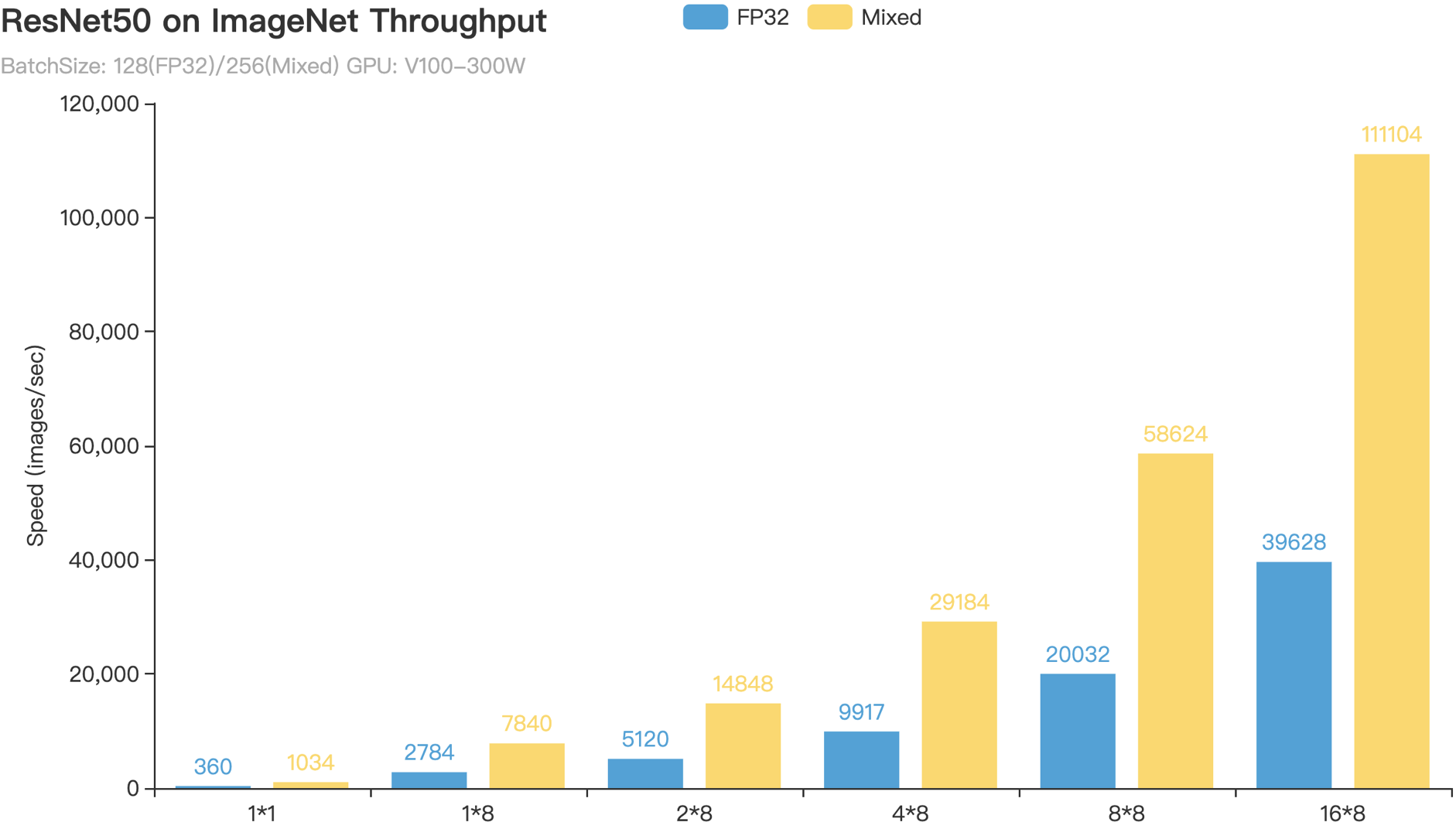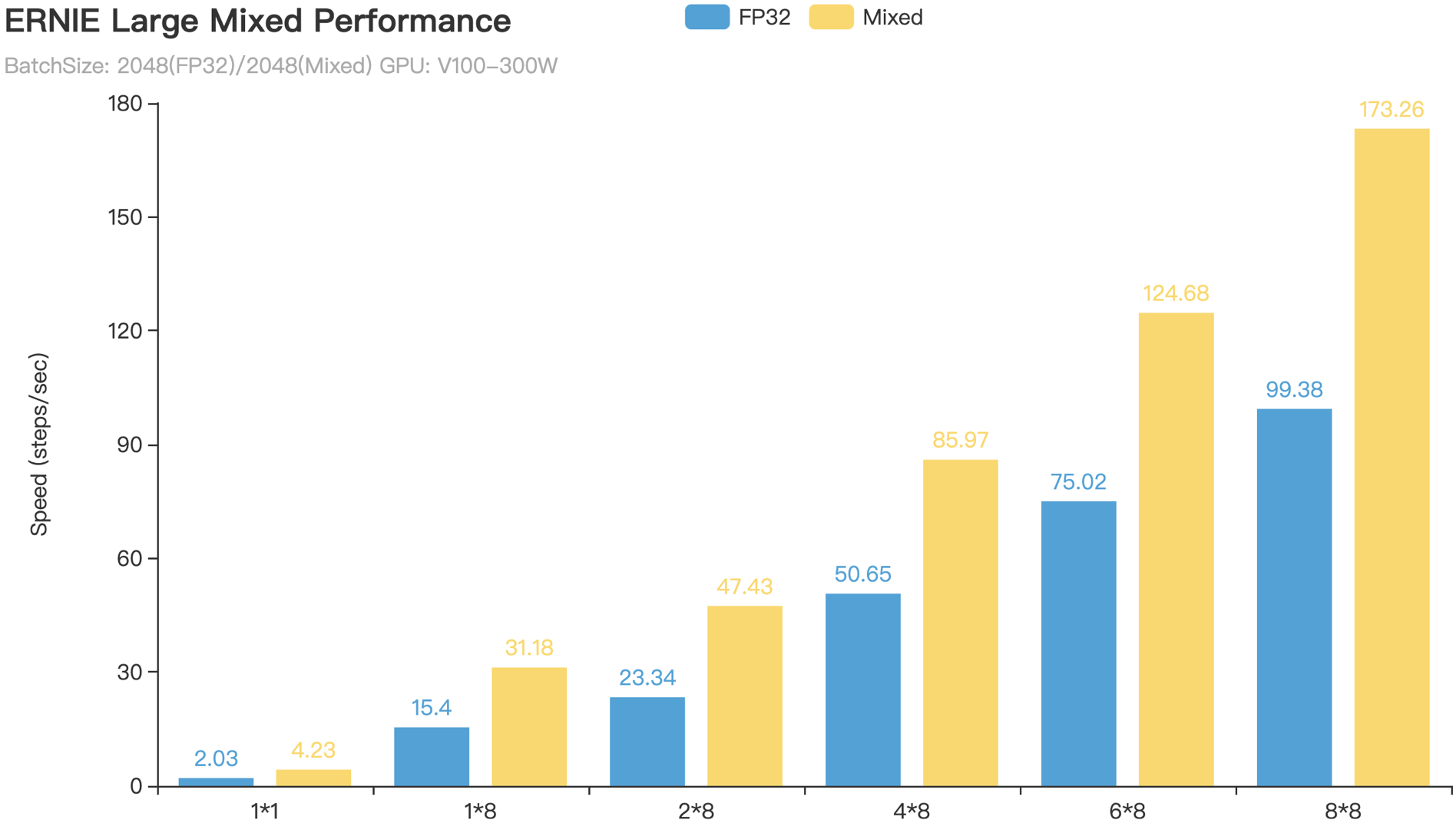# 混合精度训练最佳实践¶

Automatic Mixed Precision (AMP) 是一种自动混合使用半精度（FP16）和单精度（FP32）来加速模型训练的技术。AMP技术可方便用户快速将使用 FP32 训练的模型修改为使用混合精度训练，并通过黑白名单和动态loss scaling来保证训练时的数值稳定性进而避免梯度Infinite或者NaN(Not a Number)。借力于新一代NVIDIA GPU中Tensor Cores的计算性能，PaddlePaddle AMP技术在ResNet50、Transformer等模型上训练速度相对于FP32训练加速比可达1.5～2.9。

## 英伟达GPU的FP16算力¶

• FP16可降低一半的内存带宽和存储需求，这使得在相同的硬件条件下研究人员可使用更大更复杂的模型以及更大的batch size大小。

• FP16可以充分利用英伟达Volta及Turing架构GPU提供的Tensor Cores技术。在相同的GPU硬件上，Tensor Cores的FP16计算吞吐量是FP32的8倍。

MNIST网络定义

import paddle.fluid as fluid

def MNIST(data, class_dim):
conv1 = fluid.layers.conv2d(data, 16, 5, 1, act=None, data_format='NHWC')
bn1 = fluid.layers.batch_norm(conv1, act='relu', data_layout='NHWC')
pool1 = fluid.layers.pool2d(bn1, 2, 'max', 2, data_format='NHWC')
conv2 = fluid.layers.conv2d(pool1, 64, 5, 1, act=None, data_format='NHWC')
bn2 = fluid.layers.batch_norm(conv2, act='relu', data_layout='NHWC')
pool2 = fluid.layers.pool2d(bn2, 2, 'max', 2, data_format='NHWC')
fc1 = fluid.layers.fc(pool2, size=64, act='relu')
fc2 = fluid.layers.fc(fc1, size=class_dim, act='softmax')
return fc2


• conv2dbatch_norm以及pool2d等需要将数据布局设置为NHWC，这样有助于使用TensorCore技术加速计算过程1

• Tensor Cores要求在使用FP16加速卷积运算时conv2d的输入/输出通道数为8的倍数2，因此设计网络时推荐将conv2d层的输入/输出通道数设置为8的倍数。

• Tensor Cores要求在使用FP16加速矩阵乘运算时矩阵行数和列数均为8的倍数3，因此设计网络时推荐将fc层的size参数设置为8的倍数。

FP32 训练

import paddle
import numpy as np

data = fluid.layers.data(
name='image', shape=[None, 28, 28, 1], dtype='float32')
label = fluid.layers.data(name='label', shape=[None, 1], dtype='int64')

out = MNIST(data, class_dim=10)
loss = fluid.layers.cross_entropy(input=out, label=label)
avg_loss = fluid.layers.mean(loss)

sgd = fluid.optimizer.SGDOptimizer(learning_rate=1e-3)
sgd.minimize(avg_loss)


AMP训练

sgd = SGDOptimizer(learning_rate=1e-3)
# 此处只需要使用fluid.contrib.mixed_precision.decorate将sgd封装成AMP训练所需的
# 优化器mp_sgd，并使用mp_sgd.minimize(avg_loss)代替原来的sgd.minimize(avg_loss)语句即可。
mp_sgd = fluid.contrib.mixed_precision.decorator.decorate(sgd)
mp_sgd.minimize(avg_loss)


export FLAGS_conv_workspace_size_limit=1024 # MB，根据所使用的GPU显存容量及模型特点设置数值，值越大越有可能选择到更快的卷积算法
export FLAGS_cudnn_exhaustive_search=1 # 使用穷举搜索方法来选择快速卷积算法
export FLAGS_cudnn_batchnorm_spatial_persistent=1 # 用于触发batch_norm和relu的融合


### 自定义黑白名单¶

PaddlePaddle AMP功能实现中根据FP16数据类型计算稳定性和加速效果在框架内部定义了算子（Op）的黑白名单。具体来说，将对FP16计算友好且能利用Tensor Cores的Op归类于白名单，将使用FP16计算会导致数值不稳定的Op归类于黑名单，将对FP16计算没有多少影响的Op归类于灰名单。然而，框架开发人员不可能考虑到所有的网络模型情况，尤其是那些特殊场景中使用到的模型。用户可以在使用fluid.contrib.mixed_precision.decorate 函数时通过指定自定义的黑白名单列表来改变默认的FP16计算行为。

sgd = SGDOptimizer(learning_rate=1e-3)
# list1是白名单op列表，list2是黑名单op列表，list3是黑名单var_name列表（凡是以这些黑名单var_name为输入或输出的op均会被视为黑名单op）
amp_list = AutoMixedPrecisionLists(custom_white_list=list1, custom_black_list=list2, custom_black_varnames=list3)
mp_sgd = fluid.contrib.mixed_precision.decorator.decorate(sgd, amp_list)
mp_sgd.minimize(avg_loss)


### 自动loss scaling¶

sgd = SGDOptimizer(learning_rate=1e-3)
mp_sgd = fluid.contrib.mixed_precision.decorator.decorate(sgd,
amp_lists=None,
init_loss_scaling=2**8,
incr_every_n_steps=500,
decr_every_n_nan_or_inf=4,
incr_ratio=2.0,
decr_ratio=0.5,
use_dynamic_loss_scaling=True)
mp_sgd.minimize(avg_loss)


init_loss_scaling incr_every_n_steps 以及decr_every_n_nan_or_inf等参数控制着自动loss scaling的行为。它们仅当 use_dynamic_loss_scaling设置为True时有效。下面详述这些参数的意义：

• init_loss_scaling(float)：初始loss scaling值。

• incr_every_n_steps(int)：每经过incr_every_n_steps个连续的正常梯度值才会增大loss scaling值。

• decr_every_n_nan_or_inf(int)：每经过decr_every_n_nan_or_inf个连续的无效梯度值(nan或者inf)才会减小loss scaling值。

• incr_ratio(float)：每次增大loss scaling值的扩增倍数，其为大于1的浮点数。

• decr_ratio(float)：每次减小loss scaling值的比例系数，其为小于1的浮点数。

## 多卡GPU训练的优化¶